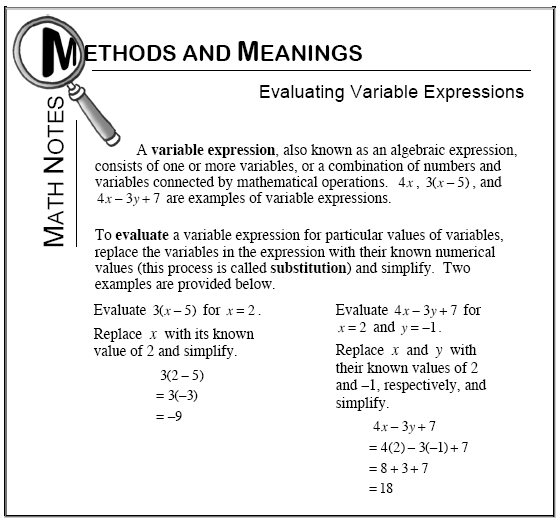### Home > MC1 > Chapter 9 > Lesson 9.2.4 > Problem9-91

9-91.

Evaluate the expressions below using $r = 3$ and $h = 5$.

1. $6h - 4$

Look at the Math Notes box below for help getting started on this problem.

$6\left(5\right) − 4 = 26$

1. $8r + h$

Remember that $8r$ means $8$ r's or$\left(8\right)\left(r\right)$.

$29$

1. $r^{2}$

Remember that the exponent (the two) means that you will multiply $r$ by itself.